# Learning JavaScript Data Structures and Algorithms5 (2 reviews total)
By Loiane Groner
• \$27.99 eBook
• \$46.99 Print + eBook
• \$12.99 eBook + Subscription
What do you get with a Packt Subscription?

• Constantly updated with 100+ new titles each month
• Breadth and depth in over 1,000+ technologies

A data structure is a particular way of organizing data in a computer to utilize resources efficiently. Data structures and algorithms are the base of every solution to any programming problem.

This book begins by covering the basics of the JavaScript language and then moves on to discuss the most important data structures such as array, queue, stack, and linked list. You will also gain an in-depth knowledge of how hash tables and set data structure function. After this, you will be taught what trees are, and how to use the binary tree and the binary search tree.

In subsequent chapters, you will learn about graphs, DFS, and BFS. Finally, we will round off by learning how to differentiate between various searching and sorting algorithms such as sequential search, binary search, quick sort, bubble sort, and so on, and how to implement them. Towards the end of the book, you will also be introduced to dynamic programming.

Publication date:
October 2014
Publisher
Packt
Pages
218
ISBN
9781783554874

## Chapter 1. JavaScript – A Quick Overview

JavaScript is a very powerful language. It is the most popular language in the world and is one of the most prominent languages on the Internet. For example, GitHub (the world's largest code host, available at https://github.com) hosts over 400,000 JavaScript repositories (the largest number of projects is in JavaScript; refer to http://goo.gl/ZFx6mg). The number of projects in JavaScript in GitHub grows every year.

JavaScript is not a language that can only be used in the frontend. It can also be used in the backend as well, and Node.js is the technology responsible for this. The number of Node Packages Modules (https://www.npmjs.org/) also grows exponentially.

JavaScript is a must-have on your résumé if you are or going to become a web developer.

In this book, you are going to learn about the most used data structures and algorithms. But why use JavaScript to learn about data structures and algorithms? We have already answered this question. JavaScript is very popular, and JavaScript is appropriate to learn about data structures because it is a functional language. Also, this can be a very fun way of learning something new, as it is very different (and easier) than learning about data structures with a standard language such as C or Java. And who said data structures and algorithms were only made for languages such as C and Java? You might need to implement some of these languages while developing for the frontend as well.

Learning about data structures and algorithms is very important. The first reason is because data structures and algorithms can solve the most common problems efficiently. This will make a difference on the quality of the source code you write in the future (including performance—if you choose the incorrect data structure or algorithm depending on the scenario, you can have some performance issues). Secondly, algorithms are studied in college together with introductory concepts of Computer Science. And thirdly, if you are planning to get a job in the greatest IT (Information Technology) companies (such as Google, Amazon, Ebay, and so on), data structures and algorithms are subjects of interview questions.

## Setting up the environment

One of the pros of the JavaScript language compared to other languages is that you do not need to install or configure a complicated environment to get started with it. Every computer has the required environment already, even though the user may never write a single line of source code. All we need is a browser!

To execute the examples in this book, it is recommended that you have Google Chrome or Firefox installed (you can use the one you like the most), an editor of your preference (such as Sublime Text), and a web server (XAMPP or any other of your preference—but this step is optional). Chrome, Firefox, Sublime Text, and XAMPP are available for Windows, Linux, and Mac OS.

If you use Firefox, it is also recommended to install the Firebug add-on (https://getfirebug.com/).

We are going to present you with three options to set up your environment.

### The browser is enough

The simplest environment that you can use is a browser.

You can use Firefox + Firebug. When you have Firebug installed, you will see the following icon in the upper-right corner:When you open Firebug (simply click on its icon), you will see the Console tab and you will be able to write all your JavaScript code on its command-line area as demonstrated in the following screenshot (to execute the source code you need to click on the Run button):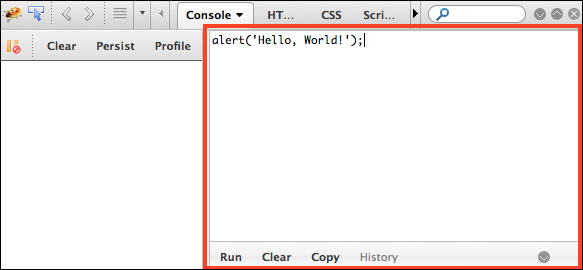You can also expand the command line to fit the entire available area of the Firebug add-on.

You can also use Google Chrome. Chrome already comes with Google Developer Tools. To open it, locate the setting and control icon and navigate to Tools | Developer Tools, as shown in the following screenshot: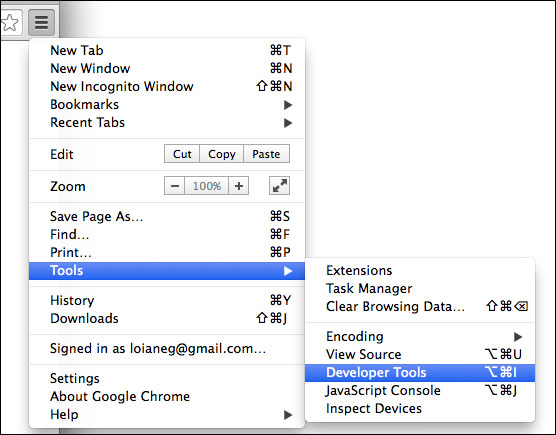Then, in the Console tab, you can write your own JavaScript code for testing, as follows: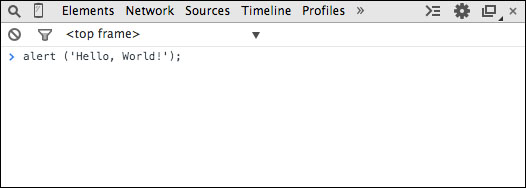### Using web servers (XAMPP)

The second environment you might want to install on your computer is also simple, but a little bit more complex than just using a browser.

You will need to install XAMPP (https://www.apachefriends.org) or any web server of your preference. Then, inside the XAMPP installation folder, you will find the `htdocs` directory. You can create a new folder where you can execute the source code we will implement in this book, or you can download the source code from this book and extract it to the `htdocs` directory, as follows: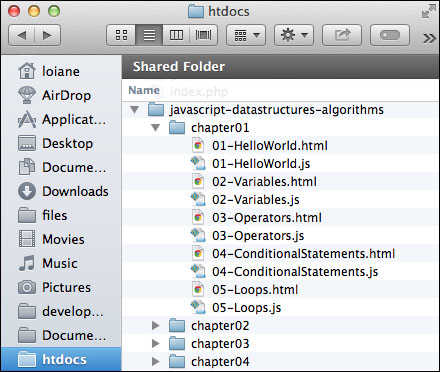Then, you can access the source code from your browser using your localhost URL (after starting the XAMPP server) as shown in the following screenshot (do not forget to enable Firebug or Google Developer Tools to see the output):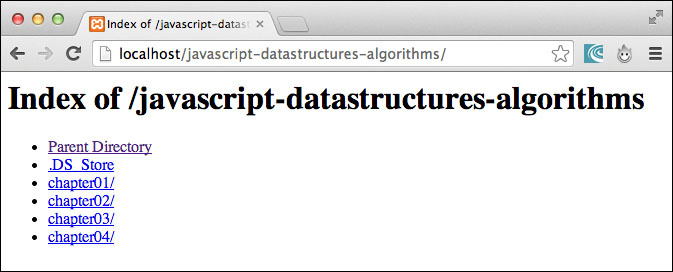### Tip

When executing the examples, always remember to have Google Developer Tools or Firebug open to see the output.

### It's all about JavaScript (Node.js)

The third option is having an environment that is 100 percent JavaScript! Instead of using XAMPP, which is an Apache server, we can use a JavaScript server.

To do so, we need to have Node.js installed. Go to http://nodejs.org/ and download and install Node.js. After that, open the terminal application (if you are using Windows, open the command prompt with Node.js that was installed with Node.js) and run the following command:

```npm install http-server –g
```

Make sure you type the command and don't copy and paste it. Copying the command might give you some errors.

You can also execute the command as an administrator. For Linux and Mac systems, use the following command:

```sudo npm install http-server –g
```

This command will install `http-server`, which is a JavaScript server. To start a server and run the examples from this book in the terminal application, change the directory to the folder that contains the book's source code and type `http-server`, as displayed in the following screenshot: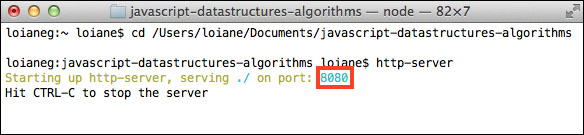To execute the examples, open the browser and access the localhost on the port specified by the `http-server` command: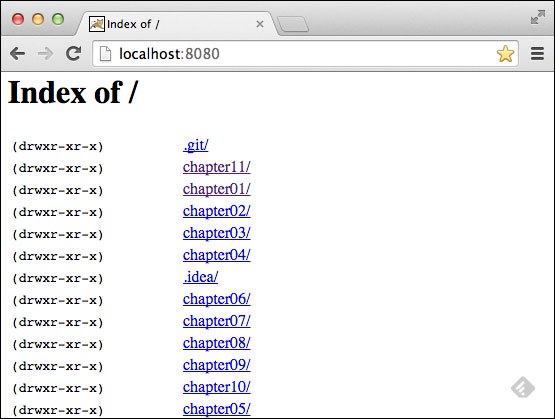### Tip

You can download the example code files for all Packt books you have purchased from your account at http://www.packtpub.com. If you purchased this book elsewhere you can visit http://www.packtpub.com/support and register to have the files e-mailed directly to you.

For this book, the code files can be downloaded from this GitHub repository: https://github.com/loiane/javascript-datastructures-algorithms.

## JavaScript basics

Before we start diving into the various data structures and algorithms, let's have a quick overview of the JavaScript language. This section will present the JavaScript basics required to implement the algorithms we will create in the subsequent chapters.

To start, let's see the two different ways we can use JavaScript code in an HTML page:

```<!DOCTYPE html>
<html>
<meta charset="UTF-8">
<body>
<script>
</script>
</body>
</html>```

The first way is demonstrated by the previous code. We need to create an HTML file and write this code on it. In this example, we are declaring the `script` tag inside the HTML file, and inside the `script` tag, we have the JavaScript code.

For the second example, we need to create a JavaScript file (we can save it as `01-HelloWorld.js`), and inside this file, we will insert the following code:

`alert('Hello, World!');`

Then, our HTML file will look like this:

```<!DOCTYPE html>
<html>
<meta charset="UTF-8">
<body>
<script src="01-HelloWorld.js">
</script>
</body>
</html>```

The second example demonstrates how to include a JavaScript file inside an HTML file.

By executing any of these two examples, the output will be the same. However, the second example is the best practice.

### Note

You may find JavaScript `include` statements or JavaScript code inside the `head` tag in some examples on the Internet. As a best practice, we will include any JavaScript code at the end of the `body` tag. This way, the HTML will be parsed by the browser and displayed before the scripts are loaded. This boosts the performance of the page.

### Variables

Variables store data that can be set, updated, and retrieved whenever needed. Values that are assigned to a variable belong to a type. In JavaScript, the available types are numbers, strings, Booleans, functions, and objects. We also have undefined and null, along with arrays, dates, and regular expressions. The following is an example of how to use variables in JavaScript:

```var num = 1; //{1}
num = 3; //{2}

var price = 1.5; //{3}
var name = 'Packt'; //{4}
var trueValue = true; //{5}
var nullVar = null; //{6}
var und;  //7```

On line `{1}`, we have an example of how to declare a variable in JavaScript (we are declaring a number). Although it is not necessary to use the `var` keyword declaration, it is a good practice to always specify when we are declaring a new variable.

On line `{2}`, we are updating an existing variable. JavaScript is not a strongly-typed language. This means you can declare a variable and initialize it with a number, and then update it with a string or any other data type. Assigning a value to a variable that is different from its original type is also not a good practice.

On line `{3}`, we are also declaring a number, but this time it is a decimal floating point. On line `{4}`, we are declaring a string; on line `{5}`, we are declaring a Boolean. On line `{6}`, we are declaring a `null` value, and on line `{7}`, we are declaring an undefined variable. A `null` value means no value and `undefined` means a variable that has been declared but not yet assigned a value:

```console.log("num: "+ num);
console.log("name: "+ name);
console.log("trueValue: "+ trueValue);
console.log("price: "+ price);
console.log("nullVar: "+ nullVar);
console.log("und: "+ und);```

If we want to see the value of each variable we have declared, we can use `console.log` to do so, as listed in the previous code snippet.

### Note

We have three ways of outputting values in JavaScript that we can use with the examples of this book. The first one is `alert('My text here')`, which will output an alert window on the browser; the second one is `console.log('My text here')`, which will output text on the Console tab of the debug tool (Google Developer Tools or Firebug, depending on the browser you are using). Finally, the third way is outputting the value directly on the HTML page that is being rendered by the browser by using `document.write('My text here')`. You can use the option that you feel most comfortable with.

The `console.log` method also accepts more than just arguments. Instead of `console.log("num: "+ num)`, we can also use `console.log("num: ", num)`.

We will discuss functions and objects later in this chapter.

#### Variable scope

Scope refers to where in the algorithm we can access the variable (it can also be a function when we are working with function scopes). There are local and global variables.

Let's look at an example:

```var myVariable = 'global';
myOtherVariable = 'global';

function myFunction(){
var myVariable = 'local';
return myVariable;
}

function myOtherFunction(){
myOtherVariable = 'local';
return myOtherVariable;
}

console.log(myVariable);   //{1}
console.log(myFunction()); //{2}

console.log(myOtherVariable);   //{3}
console.log(myOtherFunction()); //{4}
console.log(myOtherVariable);   //{5}```

Line `{1}` will output `global` because we are referring to a global variable. Line `{2}` will output `local` because we declared the `myVariable` variable inside the `myFunction` function as a local variable, so the scope will be inside `myFunction` only.

Line `{3}` will output `global` because we are referencing the global variable named `myOtherVariable` that was initialized in the second line of the example. Line `{4}` will output `local`. Inside the `myOtherFunction` function, we are referencing the `myOtherVariable` global variable and assigning the value `local` to it because we are not declaring the variable using the `var` keyword. For this reason, line `{5}` will output `local` (because we changed the value of the variable inside `myOtherFunction`).

You may hear that global variables in JavaScript are evil, and this is true. Usually, the quality of JavaScript source code is measured by the number of global variables and functions (a large number is bad). So, whenever possible, try avoiding global variables.

### Operators

We need operators when performing any operation in a programming language. JavaScript also has arithmetic, assignment, comparison, logical, bitwise, and unary operators, among others. Let's take a look at them:

```var num = 0; // {1}
num = num + 2;
num = num * 3;
num = num / 2;
num++;
num--;

num += 1; // {2}
num -= 2;
num *= 3;
num /= 2;
num %= 3;

console.log('num == 1 : ' + (num == 1)); // {3}
console.log('num === 1 : ' + (num === 1));
console.log('num != 1 : ' + (num != 1));
console.log('num > 1 : ' + (num > 1));
console.log('num < 1 : ' + (num < 1));
console.log('num >= 1 : ' + (num >= 1));
console.log('num <= 1 : ' + (num <= 1));

console.log('true && false : ' + (true && false)); // {4}
console.log('true || false : ' + (true || false));
console.log('!true : ' + (!true));```

On line `{1}`, we have the arithmetic operators. In the following table, we have the operators and their descriptions:

Arithmetic operator

Description

`+`

`-`

Subtraction

`*`

Multiplication

`/`

Division

`%`

Modulus (remainder of a division operation)

`++`

Increment

`--`

Decrement

On line `{2}`, we have the assignment operators. In the following table, we have the operators and their descriptions:

Assignment operator

Description

`=`

Assignment

`+=`

Addition assignment (x += y) == (x = x + y)

`-=`

Subtraction assignment (x -= y) == (x = x - y)

`*=`

Multiplication assignment (x *= y) == (x = x * y)

`/=`

Division assignment (x /= y) == (x = x / y)

`%=`

Remainder assignment (x %= y) == (x = x % y)

On line `{3}`, we have the comparison operators. In the following table, we have the operators and their descriptions:

Comparison operator

Description

`==`

Equal to

`===`

Equal to (value and object type both)

`!=`

Not equal to

`>`

Greater than

`>=`

Greater than or equal to

`<`

Less than

`<=`

Less than or equal to

And on line `{4}`, we have the logical operators. In the following table, we have the operators and their descriptions:

Logical operator

Description

`&&`

And

`||`

Or

`!`

Not

JavaScript also supports bitwise operators, shown as follows:

```console.log('5 & 1:', (5 & 1));
console.log('5 | 1:', (5 | 1));
console.log('~ 5:', (~5));
console.log('5 ^ 1:', (5 ^ 1));
console.log('5 << 1:', (5 << 1));
console.log('5 >> 1:', (5 >> 1));```

The following table contains more detailed descriptions of the bitwise operators:

Bitwise operator

Description

&

And

|

Or

~

Not

^

Xor

<<

Left shift

>>

Right shift

The `typeof` operator returns the type of the variable or expression. For example, have a look at the following code:

```console.log('typeof num:', typeof num);
console.log('typeof Packt:', typeof 'Packt');
console.log('typeof true:', typeof true);
console.log('typeof [1,2,3]:', typeof [1,2,3]);
console.log('typeof {name:John}:', typeof {name:'John'});```

The output will be as follows:

```typeof num: number
typeof Packt: string
typeof true: boolean
typeof [1,2,3]: object
typeof {name:John}: object```

JavaScript also supports the `delete` operator, which deletes a property from an object:

```var myObj = {name: 'John', age: 21};
delete myObj.age;
console.log(myObj); //outputs Object {name: "John"}```

In this book's algorithms, we will be using some of these operators.

### Truthy and falsy

In JavaScript, true and false are a little bit tricky. In most languages, the Boolean values `true` and `false` represent the true/false results. In JavaScript, a string suchas "Packt" has the value `true`, for example.

The following table can help us better understand how `true` and `false` work in JavaScript:

Value type

Result

`undefined`

`false`.

`null`

`false`.

`Boolean`

true is `true` and false is `false`!

`Number`

The result is `false` for +0, -0, or `NaN`; otherwise, the result is `true`.

`String`

The result is `false` if the string is empty (length is 0); otherwise, the result is `true` (length > 1).

`Object`

`true`.

Let's see some examples and verify their output:

```function testTruthy(val){
return val ? console.log('truthy') : console.log('falsy');
}

testTruthy(true); //true
testTruthy(false); //false
testTruthy(new Boolean(false)); //true (object is always true)

testTruthy(''); //false
testTruthy('Packt'); //true
testTruthy(new String('')); //true (object is always true)

testTruthy(1); //true
testTruthy(-1); //true
testTruthy(NaN); //false
testTruthy(new Number(NaN)); //true (object is always true)

testTruthy({}); //true (object is always true)

var obj = {name:'John'};
testTruthy(obj); //true
testTruthy(obj.name); //true
testTruthy(obj.age); //false (age does not exist)```

### The equals operators (== and ===)

The two equals operators supported by JavaScript can cause a little bit of confusion when working with them.

When using `==`, values can be considered equal even when they are of different types. This can be confusing even for a senior JavaScript developer. Let's analyze how `==` works using the following table:

Type(x)

Type(y)

Result

`null`

`undefined`

`true`

`undefined`

`null`

true

`Number`

`String`

`x == toNumber(y)`

`String`

`Number`

`toNumber(x) == y`

`Boolean`

`Any`

`toNumber(x) == y`

`Any`

`Boolean`

`x == toNumber(y)`

`String` or `Number`

`Object`

`x == toPrimitive(y)`

`Object`

`String` or `Number`

`toPrimitive(x) == y`

If x and y are the same type, then JavaScript will use the equals method to compare the two values or objects. Any other combination that is not listed in the table gives a false result.

The `toNumber` and `toPrimitive` methods are internal and evaluate the values according to the tables that follow.

The `toNumber` method is presented here:

Value type

Result

`undefined`

`NaN`.

`null`

+0.

`Boolean`

If the value is `true`, the result is 1; if the value is `false`, the result is +0.

`Number`

The value of the number.

`String`

This parses the string into a number. If the string consists of alphabetical characters, the result is NaN; if the string consists of numbers, it is transformed into a number.

`Object`

`toNumber(toPrimitive(value))`.

And `toPrimitive` is presented here:

Value type

Result

`Object`

If `valueOf` returns a primitive value, this returns the primitive value; otherwise, if `toString` returns a primitive value, this returns the primitive value; otherwise returns an error.

Let's verify the results of some examples. First, we know that the output of the following code is true (string length > 1):

`console.log('packt' ? true : false);`

Now, what about the following code? Let's see:

`console.log('packt' == true);`

The output is false! Let's understand why:

1. First, it converts the Boolean value using `toNumber`, so we have `packt == 1`.

2. Then, it converts the string value using `toNumber`. As the string consists of alphabetical characters, it returns NaN, so we have `NaN == 1`, which is false.

And what about the following code? Let's see:

`console.log('packt' == false);`

The output is also false! The following are the steps:

1. First, it converts the Boolean value using `toNumber`, so we have `packt == 0`.

2. Then, it converts the string value using `toNumber`. As the string consists of alphabetical characters, it returns NaN, so we have `NaN == 0`, which is false.

And what about the operator `===`? It is much easier. If we are comparing two values of different types, the result is always false. If they have the same type, they are compared according to the following table:

Type(x)

Values

Result

`Number`

x has the same value as y (but not NaN)

true

`String`

x and y are identical characters

true

`Boolean`

x and y are both true or both false

true

`Object`

x and y reference the same object

true

If x and y are different types, then the result is false.

Let's see some examples:

```console.log('packt' === true); //false

console.log('packt' === 'packt'); //true

var person1 = {name:'John'};
var person2 = {name:'John'};
console.log(person1 === person2); //false, different objects```

## Control structures

JavaScript has a similar set of control structures as the C and Java languages. Conditional statements are supported by `if…else` and `switch`. Loops are supported by `while`, `do…while`, and `for` constructs.

### Conditional statements

The first conditional statement we will take a look at is the `if…else` construct. There are a few ways we can use the `if…else` construct.

We can use the `if` statement if we want to execute a script only if the condition is `true`:

```var num = 1;
if (num === 1) {
console.log("num is equal to 1");
} ```

We can use the `if…else` statement if we want to execute a script if the condition is `true` or another script just in case the condition is `false` (`else`):

```var num = 0;
if (num === 1) {
console.log("num is equal to 1");
} else {
console.log("num is not equal to 1, the value of num is " + num);
}```

The `if…else` statement can also be represented by a ternary operator. For example, take a look at the following `if…else` statement:

```if (num === 1){
num--;
} else {
num++;
}```

It can also be represented as follows:

`(num === 1) ? num-- : num++;`

And if we have several scripts, we can use `if…else` several times to execute different scripts based on different conditions:

```var month = 5;
if (month === 1) {
console.log("January");
} else if (month === 2){
console.log("February");
} else if (month === 3){
console.log("March");
} else {
console.log("Month is not January, February or March");
}```

Finally, we have the `switch` statement. If the condition we are evaluating is the same as the previous one (however, it is being compared to different values), we can use the `switch` statement:

```var month = 5;
switch(month) {
case 1:
console.log("January");
break;
case 2:
console.log("February");
break;
case 3:
console.log("March");
break;
default:
console.log("Month is not January, February or March");
}```

One thing that is very important in a `switch` statement is the usage of `case` and `break` keywords. The `case` clause determines whether the value of `switch` is equal to the value of the `case` clause. The `break` statement stops the `switch` statement from executing the rest of the statement (otherwise, it will execute all the scripts from all `case` clauses below the matched case until a `break` statement is found in one of the `case` clauses). And finally, we have the `default` statement, which is executed by default if none of the case statements are `true` (or if the executed `case` statement does not have the `break` statement).

### Loops

Loops are very often used when we work with arrays (which is the subject of the next chapter). Specifically, we will be using the `for` loop in our algorithms.

The `for` loop is exactly the same as in C and Java. It consists of a loop counter that is usually assigned a numeric value, then the variable is compared against another value (the script inside the `for` loop is executed while this condition is true), and then the numeric value is increased or decreased.

In the following example, we have a `for` loop. It outputs the value of `i` on the console while `i` is less than 10; `i` is initiated with `0`, so the following code will output the values 0 to 9:

```for (var i=0; i<10; i++) {
console.log(i);
}```

The next loop construct we will look at is the `while` loop. The script inside the `while` loop is executed while the condition is true. In the following code, we have a variable, `i`, initiated with the value `0`, and we want the value of `i` to be outputted while `i` is less than 10 (or less than or equal to 9). The output will be the values from 0 to 9:

```var i = 0;
while(i<10)
{
console.log(i);
i++;
}```

The `do…while` loop is very similar to the `while` loop. The only difference is that in the `while` loop, the condition is evaluated before executing the script, and in the `do…while` loop, the condition is evaluated after the script is executed. The `do…while` loop ensures that the script is executed at least once. The following code also outputs the values 0 to 9:

```var i = 0;
do {
console.log(i);
i++;
} while (i<10)```

## Functions

Functions are very important when working with JavaScript. We will also use functions a lot in our examples.

The following code demonstrates the basic syntax of a function. It does not have arguments or the `return` statement:

```function sayHello() {
console.log('Hello!');
}```

To call this code, we simply use the following call:

`sayHello();`

We can also pass arguments to a function. Arguments are variables with which a function is supposed to do something. The following code demonstrates how to use arguments with functions:

```function output(text) {
console.log(text);
}```

To use this function, we can use the following code:

`output('Hello!');`

You can use as many arguments as you like, as follows:

`output('Hello!', 'Other text');`

In this case, only the first argument is used by the function and the second one is ignored.

A function can also return a value, as follows:

```function sum(num1, num2) {
return num1 + num2;
}```

This function calculates the sum of two given numbers and returns its result. We can use it as follows:

```var result = sum(1,2);
output(result);```

## Object-oriented programming

JavaScript objects are very simple collections of name-value pairs. There are two ways of creating a simple object in JavaScript. The first way is as follows:

`var obj = new Object();`

And the second way is as follows:

`var obj = {};`

We can also create an object entirely as follows:

```obj = {
name: {
first: 'Gandalf',
last: 'the Grey'
},
};```

In object-oriented programming (OOP), an object is an instance of a class. A class defines the characteristics of the object. For our algorithms and data structures, we will create some classes that will represent them. This is how we can declare a class that represents a book:

```function Book(title, pages, isbn){
this.title = title;
this.pages = pages;
this.isbn = isbn;
}```

To instantiate this class, we can use the following code:

`var book = new Book('title', 'pag',  'isbn');`

Then, we can access its attributes and update them as follows:

```console.log(book.title); //outputs the book title
book.title = 'new title'; //updates the value of the book title
console.log(book.title); //outputs the updated value```

A class can also contain functions. We can declare and use a function as the following code demonstrates:

```Book.prototype.printTitle = function(){
console.log(this.title);
};
book.printTitle();```

We can declare functions directly inside the class definition as well:

```function Book(title, pages, isbn){
this.title = title;
this.pages = pages;
this.isbn = isbn;
this.printIsbn = function(){
console.log(this.isbn);
}
}
book.printIsbn();```

### Note

In the prototype example, the `printTitle` function is going to be shared between all instances, and only one copy is going to be created. When we use class-based definition, as in the previous example, each instance will have its own copy of the functions. Using the prototype method saves memory and processing cost in regards to assigning the functions to the instance. However, you can only declare public functions and properties using the prototype method. With a class-based definition, you can declare private functions and properties and the other methods inside the class can also access them. You will notice in the examples of this book that we use a class-based definition (because we want to keep some properties and functions private). But, whenever possible, we should use the prototype method.

Now we have covered all the basic JavaScript concepts that are needed for us to start having some fun with data structures and algorithms!

## Debugging and tools

Knowing how to program with JavaScript is important, but so is knowing how to debug your code. Debugging is very useful to help find bugs in your code, but it can also help you execute your code at a lower speed so you can see everything that is happening (the stack of methods called, variable assignment, and so on). It is highly recommended that you spend some time debugging the source code of this book to see every step of the algorithm (it might help you understand it better as well).

Both Firefox and Chrome support debugging. A great tutorial from Google that shows you how to use Google Developer Tools to debug JavaScript can be found at https://developer.chrome.com/devtools/docs/javascript-debugging.

You can use any text editor of your preference. But there are other great tools that can help you be more productive when working with JavaScript as well:

• Aptana: This is a free and open source IDE that supports JavaScript, CSS3, and HTML5, among other languages (http://www.aptana.com/).

• WebStorm: This is a very powerful JavaScript IDE with support for the latest web technologies and frameworks. It is a paid IDE, but you can download a 30-day trial version (http://www.jetbrains.com/webstorm/).

• Sublime Text: This is a lightweight text editor, and you can customize it by installing plugins. You can buy the license to support the development team, but you can also use it for free (the trial version does not expire) at http://www.sublimetext.com/.

## Summary

In this chapter, we learned how to set up the development environment to be able to create or execute the examples in this book.

We also covered the basics of the JavaScript language that are needed prior to getting started with constructing the algorithms and data structures covered in this book.

In the next chapter, we will look at our first data structure, which is array, the most basic data structure that many languages support natively, including JavaScript.